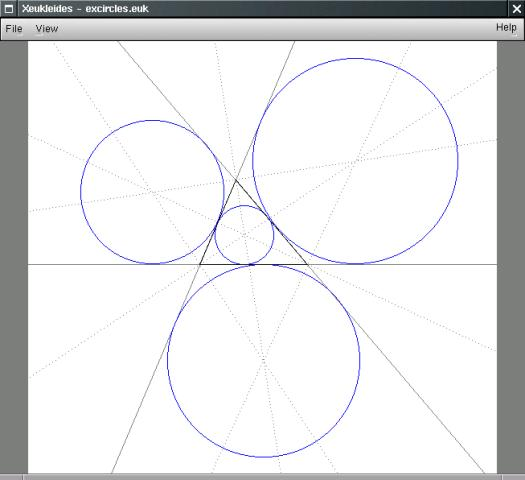## 34 Search Results

### cdd/cddplus

The program cdd+ is a C++ implementation of the Double Description Method of Motzkin et al. for generating all vertices (i.e. extreme points) and extreme rays of a general convex polyhedron in R^d given by a system of linear inequalities.

### Convex

Convex is a Maple package for convex geometry. It can deal with rational polytopes, cones and other polyhedra of arbitrary dimension. Polyhedral complexes and fans are also supported, as well as computations in face lattices. The integration into Maple makes Convex particularly suited for "applied" problems where polyhedra arise together with other mathematical structures.

### ePix

ePiX, a collection of batch-oriented utilities for *nix, creates mathematically accurate line figures, plots, and movies using easy-to-learn syntax. LaTeX and dvips comprise the typographical rendering engine, while ImageMagick is used to create bitmapped images and animations. The user interface resembles that of LaTeX itself: You prepare a short scene description in a text editor, then compile'' the input file into a picture. Default output formats are eepic (a plain text enhancement to the LaTeX picture environment), eps, pdf, png, and mng.

More information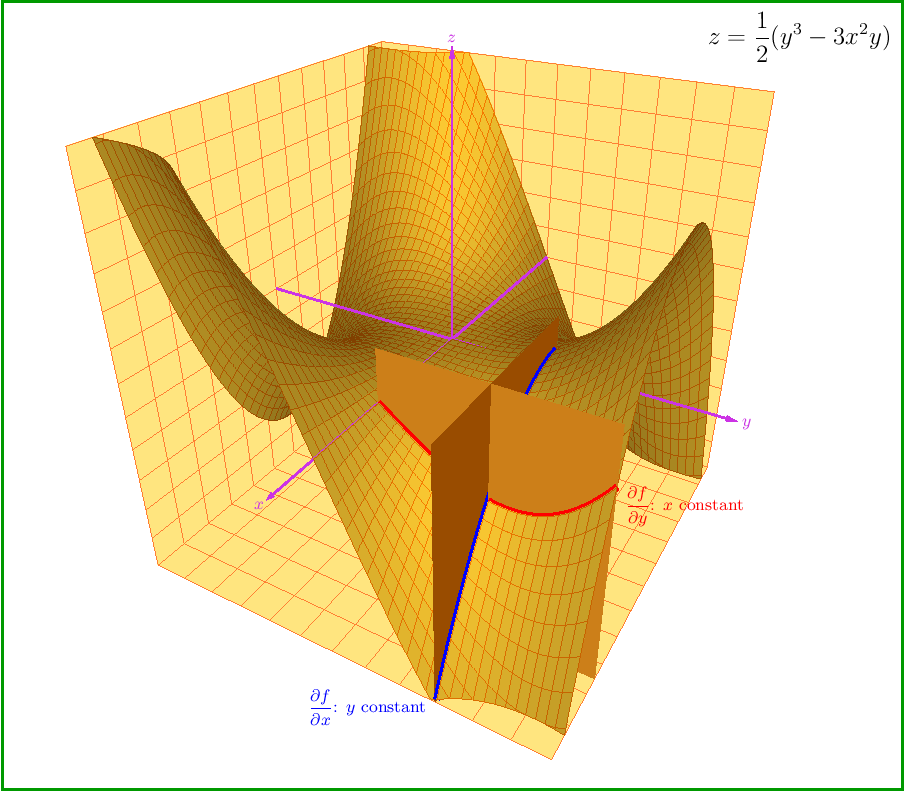### FLINT: Fast Library for Number Theory

FLINT is a C library for doing number theory.

### GAP

GAP is a system for computational discrete algebra, with particular emphasis on Computational Group Theory. GAP provides a programming language, a library of thousands of functions implementing algebraic algorithms written in the GAP language as well as large data libraries of algebraic objects. GAP is used in research and teaching for studying groups and their representations, rings, vector spaces, algebras, combinatorial structures, and more. GAP is developed by international cooperation. The system, including source, is distributed freely under the terms of the GNU General Public License. You can study and easily modify or extend GAP for your special use. The current version is GAP 4, the older version GAP 3 is still available.

### GeoGebra

GeoGebra is free and multi-platform dynamic mathematics software for all levels of education that joins geometry, algebra, tables, graphing, statistics and calculus in one easy-to-use package. It has received several educational software awards in Europe and the USA.Quick Facts: * Graphics, algebra and tables are connected and fully dynamic * Easy-to-use interface, yet many powerful features * Authoring tool to create interactive learning materials as web pages * Available in many languages for our millions of users around the world * Free and open source software

More information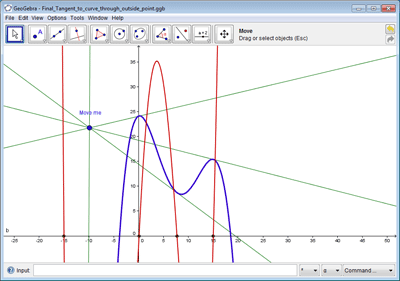### GiANT

GiANT is a graphical interface for working with number fields. It dynamically provides the user with typeset information, diagrams, and drag-and-drop functionality. The result is a level of human-computer interaction which is difficult to achieve in a shell environment. GiANT is written in Java 1.4 and runs on top of the computer algebra system KASH 2.5.

### Givaro

In the joint CNRS-INRIA / INPG-UJF project APACHE, Givaro is a C++ library for arithmetic and algebraic computations. Its main features are implementations of the basic arithmetic of many mathematical entities: Primes fields, Extensions Fields, Finite Fields, Finite Rings, Polynomials, Algebraic numbers, Arbitrary precision integers and rationals (C++ wrappers over gmp) It also provides data-structures and templated classes for the manipulation of basic algebraic objects, such as vectors, matrices (dense, sparse, structured), univariate polynomials (and therefore recursive multivariate). It contains different program modules and is fully compatible with the LinBox linear algebra library and the Athapascan environment, which permits parallel programming.

### gretl - Gnu Regression, Econometrics and Time-series Library

Gretl is a statistical package. It was developed mainly for econometrics. It has as well scripting capabilities as an easy intuitive interface. It features a wide variety of estimators, time series methods limited dependent variables. It has several times been applied in the Journal of Applied Econometrics.

### igraph

igraph is a collection of network analysis tools with the emphasis on efficiency, portability and ease of use. igraph is a free and open source software package for creating and manipulating undirected and directed graphs. It includes implementations for classic graph theory problems like minimum spanning trees and network flow, and also implements algorithms for some recent network analysis methods, like community structure search. The efficient implementation of igraph allows it to handle graphs with millions of vertices and edges. The rule of thumb is that if your graph fits into the physical memory then igraph can handle it. igraph can be programmed in R, Python and C/C++ by virtue of R/igraph, python-igraph and C/igraph, respectively. There is also a Mathematica interface IGraph/M written by Szabolcs Horvát.

### IML - Integer Matrix Library

IML is a free library of C source code which implements algorithms for computing exact solutions to dense systems of linear equations over the integers. IML is designed to be used with the ATLAS/BLAS library and GMP bignum library. Written in portable C, IML can be used on both 32-bit and 64-bit machines. It can be called from C++.

### JMulTi

JMulTi is an interactive software designed for univariate and multivariate time series analysis. It has a Java graphical user interface that uses an external engine for statistical computations. It has been designed for certain econometric procedures in time series analysis, especially some of them not available in other packages, like Impulse Response Analysis with bootstrapped confidence intervals for VAR/VEC modelling.

### LattE integrale

LattE (Lattice point Enumeration) is a computer software dedicated to the problems of counting lattice points and integrationinside convex polytopes. LattE contains the first ever implementation of Barvinok's algorithm. The latest version, LattE integrale, has the ability to directly compute integrals of polynomial functions over polytopes and in particular to do exact volume computations. Version 1.6 added the capability of computing the highest coefficients of weighted Ehrhart quasipolynomials.

### M4RI

M4RI is a library for fast arithmetic with dense matrices over F2.

More information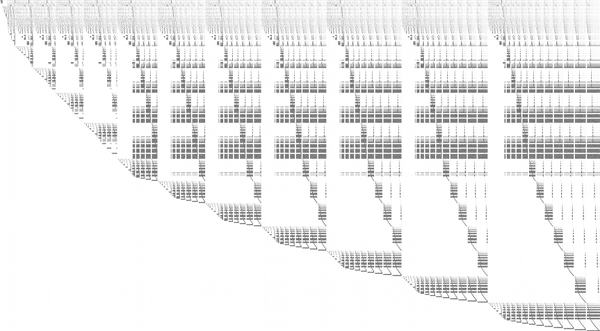### Maxima

Maxima is a system for the manipulation of symbolic and numerical expressions, including differentiation, integration, Taylor series, Laplace transforms, ordinary differential equations, systems of linear equations, and vectors, matrices, and tensors. Maxima produces high precision results by using exact fractions and arbitrarily long floating point representations, and can plot functions and data in two and three dimensions.

### Normaliz

Normaliz is a tool for computations in affine monoids, vector configurations, lattice polytopes, and rational cones. Its input data can be specified in terms of a system of generators or vertices or a system of linear homogeneous Diophantine equations, inequalities and congruences or a binomial ideal. Normaliz computes the dual cone of a rational cone (in other words, given generators, Normaliz computes the defining hyperplanes, and vice versa), a placing (or lexicographic) triangulation of a vector configuration (resulting in a triangulation of the cone generated by it), the Hilbert basis of a rational cone, the lattice points of a lattice polytope, the normalization of an affine monoid, the Hilbert (or Ehrhart) series and the Hilbert (or Ehrhart) (quasi) polynomial under a Z-grading (for example, for rational polytopes), NEW: generalized (or weighted) Ehrhart series and Lebesgue integrals of polynomials over rational polytopes via NmzIntegrate, a description of the cone and lattice under consideration by a system of inequalities, equations and congruences

### NTL

NTL is a high-performance, portable C++ library providing data structures and algorithms for manipulating signed, arbitrary length integers, and for vectors, matrices, and polynomials over the integers and over finite fields.

### Octave

GNU Octave is a high-level language, primarily intended for numerical computations. It provides a convenient command line interface for solving linear and nonlinear problems numerically, and for performing other numerical experiments using a language that is mostly compatible with Matlab. It may also be used as a batch-oriented language. Octave has extensive tools for solving common numerical linear algebra problems, finding the roots of nonlinear equations, integrating ordinary functions, manipulating polynomials, and integrating ordinary differential and differential-algebraic equations. It is easily extensible and customizable via user-defined functions written in Octave's own language, or using dynamically loaded modules written in C++, C, Fortran, or other languages.

### PLTMG

PLTMG is a package for solving elliptic partial differential equations in general regions of the plane. It is based on continuous piecewise linear triangular finite elements, and features adaptive local mesh refinement, multigraph iteration, and pseudo-arclength continuation options for parameter dependencies. It also provides options for solving several classes of optimal control and obstacle problems. The package includes an initial mesh generator and several graphics packages. Support for the Bank-Holst parallel adaptive meshing strategy is also provided. PLTMG is provided as Fortran (and a little C) source code, in both single and double precision versions. The code has interfaces to X-Windows, MPI, and Michael Holst's OpenGL image viewer SG. The X-Windows, MPI, and SG interfaces require libraries that are NOT provided as part of the PLTMG package.

### PolyBoRi

The core of PolyBoRi is a C++ library, which provides high-level data types for Boolean polynomials and monomials, exponent vectors, as well as for the underlying polynomial rings and subsets of the powerset of the Boolean variables. As a unique approach, binary decision diagrams are used as internal storage type for polynomial structures. On top of this C++-library we provide a Python interface. This allows parsing of complex polynomial systems, as well as sophisticated and extendable strategies for Gröbner base computation. PolyBoRi features a powerful reference implementation for Gröbner basis computation.

More information### polymake

polymake is an object-oriented system for experimental discrete mathematics. The typical working cycle of a polymake user starts with the construction of an object of interest, auch as a convex polytope, a finite simplicial complex, a graph, etc. It is then possible to ask the system for some of the object's properties or for some form of visualization. Further steps might include more elaborate constructions based on previously defined objects. Each class of polymake objects comes with a set of rules which describe how a new property of an object can be derived from previously known ones. It is a key feature that the user can extend or modify the set of rules, add further properties or even new classes of objects (with entirely new rule bases). The functions provided include: several convex hull algorithms, face lattices of convex polytopes, Voronoi diagrams and Delaunay decompositions (in arbitrary dimensions), simplicial homology (with integer coefficients), simplicial cup and cap products, intersection forms of triangulated 4-manifolds. Several forms of (interactive) visualization via interfaces to Geomview, JavaView and other programs.

### PSPP

PSPP is a program for statistical analysis of sampled data. It is a Free replacement for the proprietary program SPSS.

### QPA = Quivers and Path Algebras

QPA provides software for doing computations over finite dimensional quotients of path algebras. QPA has data structures for quivers, quotients of path algebras, and modules, homomorphisms and complexes of modules over quotients of path algebras. It has implementations for computing homomorphism spaces, projective resolutions, extension groups, generators of Ext-algebras, almost split sequences and more.

### R

R is a language and environment for statistical computing and graphics. It is a GNU project which is similar to the S language and environment which was developed at Bell Laboratories (formerly AT&T, now Lucent Technologies) by John Chambers and colleagues. R can be considered as a different implementation of S. There are some important differences, but much code written for S runs unaltered under R. R provides a wide variety of statistical (linear and nonlinear modelling, classical statistical tests, time-series analysis, classification, clustering, ...) and graphical techniques, and is highly extensible. The S language is often the vehicle of choice for research in statistical methodology, and R provides an Open Source route to participation in that activity. One of R's strengths is the ease with which well-designed publication-quality plots can be produced, including mathematical symbols and formulae where needed. Great care has been taken over the defaults for the minor design choices in graphics, but the user retains full control.

### RCWA

RCWA is a package for the computer algebra system GAP. It provides implementations of algorithms and methods for computing in certain infinite permutation groups. The class of groups which RCWA in principle can deal with includes the finite groups, the free groups of finite rank, the free products of finitely many finite groups, certain infinite simple groups, certain divisible torsion groups and groups of many further types. It is closed under taking direct products and under taking wreath products with finite groups and with the infinite cyclic group (Z,+).

### Regina

Regina is a suite of mathematical software for 3-manifold topologists. It focuses upon the study of 3-manifold triangulations and includes support for normal surfaces and angle structures.

More information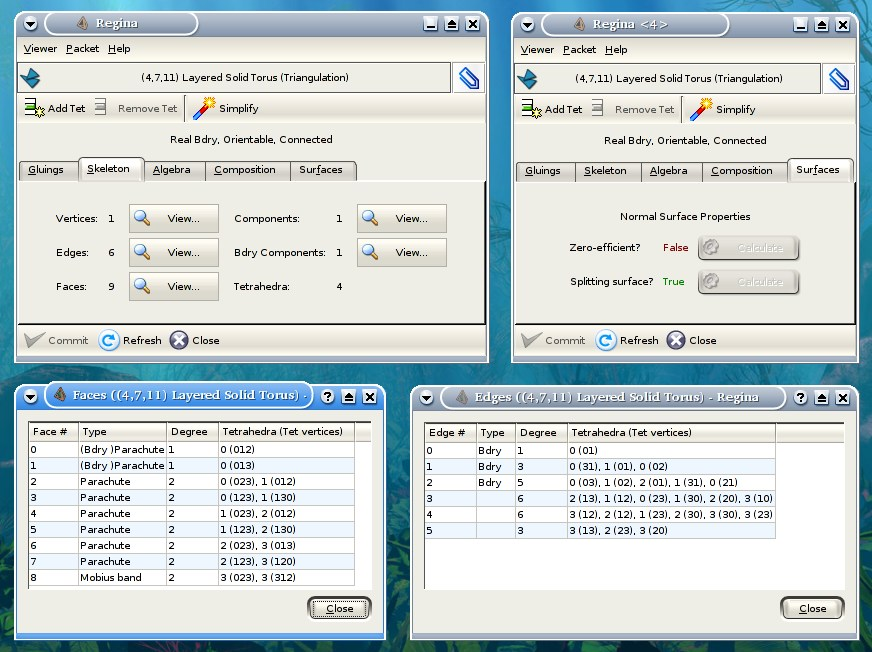### Sage

SAGE is a framework for number theory, algebra, and geometry computation. It is open source and freely available under the terms of the GNU General Public License (GPL). SAGE is a Python library with a customized interpreter. It is written in Python, C++, and C (via Pyrex). Python (http://www.python.org) is an open source object-oriented interpreted language, with a large number of libraries, e.g., for numerical analysis, which are available to users of SAGE. Python can also be accessed in library mode from C/C++ programs. SAGE provides an interface to several important open source libraries, including Cremona’s MWRANK library for computing with elliptic curves, the PARI library (pari.math.u-bordeaux.fr) for number theory, Shoup’s number theory library NTL (http://www.shoup.net/ntl/), SINGULAR (http://www.singular.uni-kl.de) for commutative algebra, GAP (http://www.gap-system.org) for group theory and combinatorics, and maxima (http://maxima.sourceforge.net) for symbolic computation and calculus.

More information### Scilab Image Processing Toolbox

SIP provides image processing, pattern recognition, and computer vision routines for SciLab, a Matlab-like matrix-oriented programming environment. SIP is able to read/write images in almost 90 major formats, including JPEG, PNG, BMP, GIF, FITS, and TIFF. It includes routines for filtering, segmentation, edge detection, morphology, curvature, fractal dimension, distance transforms, multiscale skeletons, and more.

### ShearLab 3D

ShearLab 3D is a MATLAB Library, developed for processing two- and three-dimensional signals using a certain class of basis functions named shearlets. These functions were developed with the goal of constructing systems of basis-functions nicely suited for representing anisotropic features (e.g. curvilinear singularities) that often are present in multivariate data. ShearLab 3D is optimized for GPU computing with CUDA and contains examples for applications like image and video denoising or image and video inpainting.

More information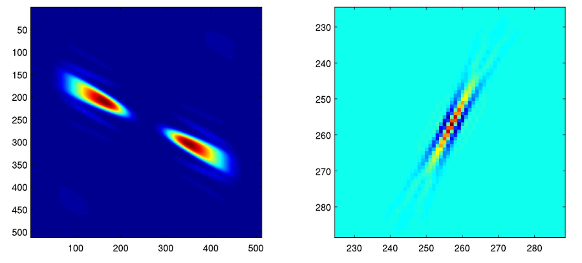### SingSurf

An online Java applet for calculation of singular algebraic surfaces.

### SINGULAR

SINGULAR is a Computer Algebra system for polynomial computations in commutative algebra, algebraic geometry, and singularity theory. SINGULAR's main computational objects are ideals and modules over a large variety of baserings. The baserings are polynomial rings over a field (e.g., finite fields, the rationals, floats, algebraic extensions, transcendental extensions), or localizations thereof, or quotient rings with respect to an ideal. SINGULAR features fast and general implementations for computing Groebner and standard bases, including e.g. Buchberger's algorithm and Mora's Tangent Cone algorithm. Furthermore, it provides polynomial factorizations, resultant, characteristic set and gcd computations, syzygy and free-resolution computations, and many more related functionalities. Based on an easy-to-use interactive shell and a C-like programming language, SINGULAR's internal functionality is augmented and user-extendible by libraries written in the SINGULAR programming language. A general and efficient implementation of communication links allows SINGULAR to make its functionality available to other programs.

### Surfer

Surfer is a programme to visualize real algebraic surfaces in an intuitive and fast way

More information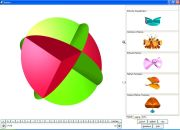### XaoS

XaoS is a fast, portable, real-time, and interactive fractal zoomer. It displays the Mandelbrot set (among other escape time fractals) and allows you zoom smoothly into the fractal. Various coloring modes are provided for both the points inside and outside the selected set. In addition, switching between Julia and Mandelbrot fractal types and on-the-fly plane switching is provided.

More information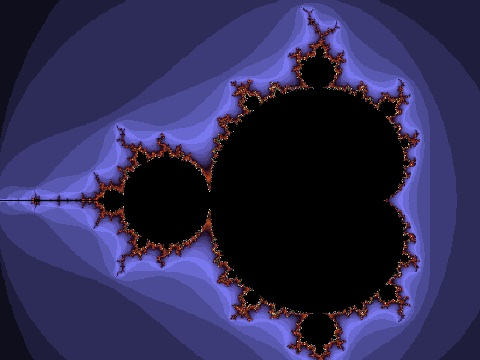### Xeukleides

Xeukleides is a frontend for editing and viewing figures described by the language Eukleides (which provides simple and powerful commands for creating elementary Euclidean geometry figures).

More information